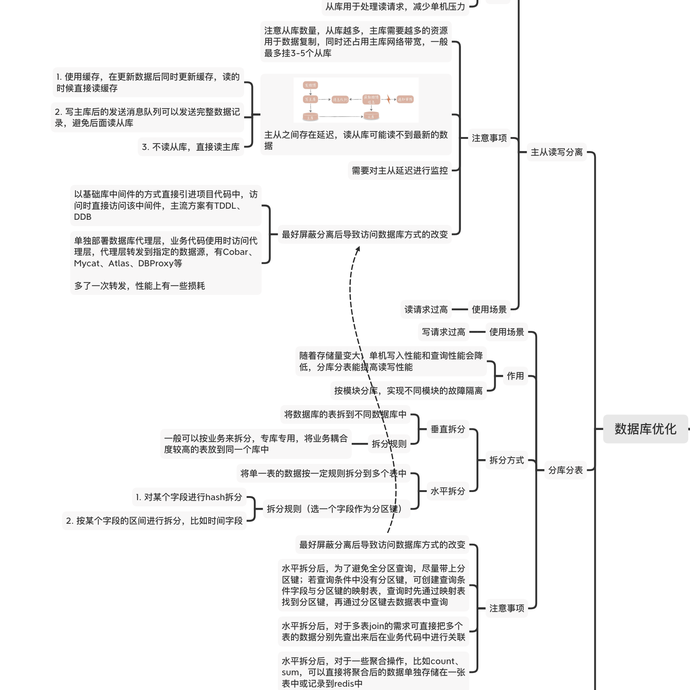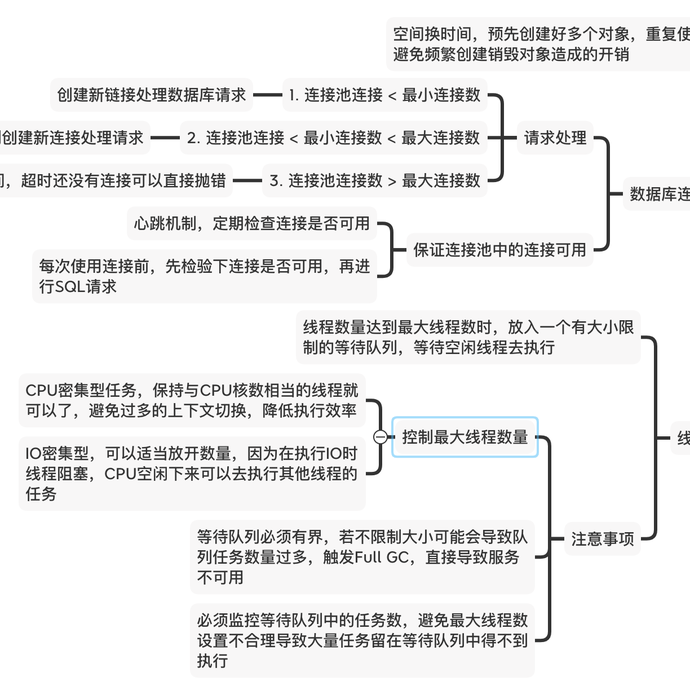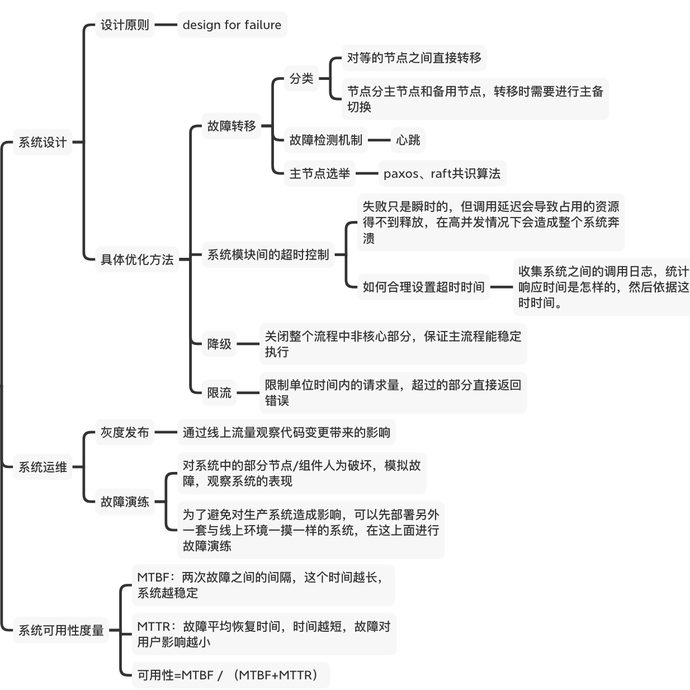0

522

design for failure

paxos、raft共识算法

MTBF：两次故障之间的间隔，这个时间越长，系统越稳定
MTTR：故障平均恢复时间，时间越短，故障对用户影响越小【高并发学习笔记】

1. 横向扩展和纵向扩展

2. 使用缓存
3. 异步处理

【面试训练】leetcode42. 给定 n 个非负整数表示每个宽度为 1 的柱子的高度图，计算按此排列的柱子，下雨之后能接多少雨水。

class Solution {
public:
int trap(vector<int>& height) {
int res = 0;
int size = height.size();
vector<int>l_max_memo(size), r_max_memo(size);

// 构建备忘录
l_max_memo = height;
r_max_memo[size - 1] = height[size - 1];
for (int i = 1; i < size; i++) {
l_max_memo[i] = height[i] > l_max_memo[i-1] ? height[i] : l_max_memo[i-1];
}

for (int i = size - 2; i >=0; i--) {
r_max_memo[i] = height[i] > r_max_memo[i+1] ? height[i] : r_max_memo[i+1];
}

for (int i = 0; i < height.size(); i++) {
int max_able_height = l_max_memo[i] > r_max_memo[i] ? r_max_memo[i] : l_max_memo[i];
res += (max_able_height - height[i]);
}
return res;
}
};

【面试有感】微信后端一面卒。。。

0

7